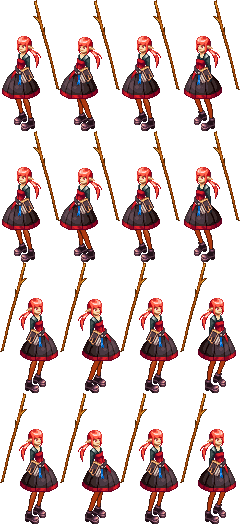# Ubuntu下使用Python实现游戏制作中的切分图片功能

更新时间：2018年03月30日 10:12:06   作者：剑有偏锋我要评论

whywhat

how

1 ubuntu下图片处理软件 GIMP： 画好参考线后， 点击 滤镜->WEB ->切片

2 python + PIL  （`pip install pillow` 安装）

```# coding=utf-8
from PIL import Image
import os
def mkdir(path):
# 去除首位空格
path=path.strip()
# 去除尾部 \ 符号
path=path.rstrip("\\")
# 判断路径是否存在
# 存在   True
# 不存在  False
isExists=os.path.exists(path)
# 判断结果
if not isExists:
# 如果不存在则创建目录
print path+' 创建成功'
# 创建目录操作函数
os.makedirs(path)
return True
else:
# 如果目录存在则不创建，并提示目录已存在
print path+' 目录已存在'
return False
cnt = 0
imageName = 'mageStand.png'
pathName = 'mageStand'
img = Image.open(imageName)
ori_w,ori_h = img.size
row = 4
col = 4
for j in range(0, col):
Y = j*ori_h/col
Y_end = Y + ori_h/col
for i in range(0, row):
X = i*ori_w/row
X_end = X + ori_w/row
print X, X_end
if 8 == cnt:
pathName+="adv"
cnt = 0
mkdir(pathName)
fileName = '%s/a_%d.png' %(pathName, cnt)
img.crop((X, Y, X_end, Y_end)).save( fileName )
cnt+=1

```